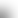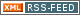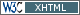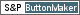www.LinuxHowtos.org howtos, tips&tricks and tutorials for linuxfrom small one page howto to huge articles all in one place

search text in:

Poll
Which linux distribution do you use?

poll results

using iotop to find disk usage hogs

using iotop to find disk usage hogs

words:

887

views:

155215

userrating:

average rating: 1.8 (88 votes) (1=very good 6=terrible)

May 25th. 2007:
Words

486

Views

246545

Workaround and fixes for the current Core Dump Handling vulnerability affected kernels

Workaround and fixes for the current Core Dump Handling vulnerability affected kernels

words:

161

views:

129753

userrating:

average rating: 1.3 (28 votes) (1=very good 6=terrible)

April, 26th. 2006:

You are here: manpages

EC_POINT_new

Section: OpenSSL (3)
Updated: 2017-05-25

NAME

EC_POINT_new, EC_POINT_free, EC_POINT_clear_free, EC_POINT_copy, EC_POINT_dup, EC_POINT_method_of, EC_POINT_set_to_infinity, EC_POINT_set_Jprojective_coordinates, EC_POINT_get_Jprojective_coordinates_GFp, EC_POINT_set_affine_coordinates_GFp, EC_POINT_get_affine_coordinates_GFp, EC_POINT_set_compressed_coordinates_GFp, EC_POINT_set_affine_coordinates_GF2m, EC_POINT_get_affine_coordinates_GF2m, EC_POINT_set_compressed_coordinates_GF2m, EC_POINT_point2oct, EC_POINT_oct2point, EC_POINT_point2bn, EC_POINT_bn2point, EC_POINT_point2hex, EC_POINT_hex2point - Functions for creating, destroying and manipulating EC_POINT objects.

SYNOPSIS

#include <openssl/ec.h>
#include <openssl/bn.h>

EC_POINT *EC_POINT_new(const EC_GROUP *group);
void EC_POINT_free(EC_POINT *point);
void EC_POINT_clear_free(EC_POINT *point);
int EC_POINT_copy(EC_POINT *dst, const EC_POINT *src);
EC_POINT *EC_POINT_dup(const EC_POINT *src, const EC_GROUP *group);
const EC_METHOD *EC_POINT_method_of(const EC_POINT *point);
int EC_POINT_set_to_infinity(const EC_GROUP *group, EC_POINT *point);
int EC_POINT_set_Jprojective_coordinates_GFp(const EC_GROUP *group, EC_POINT *p,
const BIGNUM *x, const BIGNUM *y, const BIGNUM *z, BN_CTX *ctx);
int EC_POINT_get_Jprojective_coordinates_GFp(const EC_GROUP *group,
const EC_POINT *p, BIGNUM *x, BIGNUM *y, BIGNUM *z, BN_CTX *ctx);
int EC_POINT_set_affine_coordinates_GFp(const EC_GROUP *group, EC_POINT *p,
const BIGNUM *x, const BIGNUM *y, BN_CTX *ctx);
int EC_POINT_get_affine_coordinates_GFp(const EC_GROUP *group,
const EC_POINT *p, BIGNUM *x, BIGNUM *y, BN_CTX *ctx);
int EC_POINT_set_compressed_coordinates_GFp(const EC_GROUP *group, EC_POINT *p,
const BIGNUM *x, int y_bit, BN_CTX *ctx);
int EC_POINT_set_affine_coordinates_GF2m(const EC_GROUP *group, EC_POINT *p,
const BIGNUM *x, const BIGNUM *y, BN_CTX *ctx);
int EC_POINT_get_affine_coordinates_GF2m(const EC_GROUP *group,
const EC_POINT *p, BIGNUM *x, BIGNUM *y, BN_CTX *ctx);
int EC_POINT_set_compressed_coordinates_GF2m(const EC_GROUP *group, EC_POINT *p,
const BIGNUM *x, int y_bit, BN_CTX *ctx);
size_t EC_POINT_point2oct(const EC_GROUP *group, const EC_POINT *p,
point_conversion_form_t form,
unsigned char *buf, size_t len, BN_CTX *ctx);
int EC_POINT_oct2point(const EC_GROUP *group, EC_POINT *p,
const unsigned char *buf, size_t len, BN_CTX *ctx);
BIGNUM *EC_POINT_point2bn(const EC_GROUP *, const EC_POINT *,
point_conversion_form_t form, BIGNUM *, BN_CTX *);
EC_POINT *EC_POINT_bn2point(const EC_GROUP *, const BIGNUM *,
EC_POINT *, BN_CTX *);
char *EC_POINT_point2hex(const EC_GROUP *, const EC_POINT *,
point_conversion_form_t form, BN_CTX *);
EC_POINT *EC_POINT_hex2point(const EC_GROUP *, const char *,
EC_POINT *, BN_CTX *);

DESCRIPTION

An EC_POINT represents a point on a curve. A new point is constructed by calling the function EC_POINT_new and providing the group object that the point relates to.

EC_POINT_free frees the memory associated with the EC_POINT.

EC_POINT_clear_free destroys any sensitive data held within the EC_POINT and then frees its memory.

EC_POINT_copy copies the point src into dst. Both src and dst must use the same EC_METHOD.

EC_POINT_dup creates a new EC_POINT object and copies the content from src to the newly created EC_POINT object.

EC_POINT_method_of obtains the EC_METHOD associated with point.

A valid point on a curve is the special point at infinity. A point is set to be at infinity by calling EC_POINT_set_to_infinity.

The affine co-ordinates for a point describe a point in terms of its x and y position. The functions EC_POINT_set_affine_coordinates_GFp and EC_POINT_set_affine_coordinates_GF2m set the x and y co-ordinates for the point p defined over the curve given in group.

As well as the affine co-ordinates, a point can alternatively be described in terms of its Jacobian projective co-ordinates (for Fp curves only). Jacobian projective co-ordinates are expressed as three values x, y and z. Working in this co-ordinate system provides more efficient point multiplication operations. A mapping exists between Jacobian projective co-ordinates and affine co-ordinates. A Jacobian projective co-ordinate (x, y, z) can be written as an affine co-ordinate as (x/(z^2), y/(z^3)). Conversion to Jacobian projective to affine co-ordinates is simple. The co-ordinate (x, y) is mapped to (x, y, 1). To set or get the projective co-ordinates use EC_POINT_set_Jprojective_coordinates_GFp and EC_POINT_get_Jprojective_coordinates_GFp respectively.

Points can also be described in terms of their compressed co-ordinates. For a point (x, y), for any given value for x such that the point is on the curve there will only ever be two possible values for y. Therefore a point can be set using the EC_POINT_set_compressed_coordinates_GFp and EC_POINT_set_compressed_coordinates_GF2m functions where x is the x co-ordinate and y_bit is a value 0 or 1 to identify which of the two possible values for y should be used.

In addition EC_POINTs can be converted to and from various external representations. Supported representations are octet strings, BIGNUMs and hexadecimal. Octet strings are stored in a buffer along with an associated buffer length. A point held in a BIGNUM is calculated by converting the point to an octet string and then converting that octet string into a BIGNUM integer. Points in hexadecimal format are stored in a NULL terminated character string where each character is one of the printable values 0-9 or A-F (or a-f).

The functions EC_POINT_point2oct, EC_POINT_oct2point, EC_POINT_point2bn, EC_POINT_bn2point, EC_POINT_point2hex and EC_POINT_hex2point convert from and to EC_POINTs for the formats: octet string, BIGNUM and hexadecimal respectively.

The function EC_POINT_point2oct must be supplied with a buffer long enough to store the octet string. The return value provides the number of octets stored. Calling the function with a NULL buffer will not perform the conversion but will still return the required buffer length.

The function EC_POINT_point2hex will allocate sufficient memory to store the hexadecimal string. It is the caller's responsibility to free this memory with a subsequent call to OPENSSL_free().

RETURN VALUES

EC_POINT_new and EC_POINT_dup return the newly allocated EC_POINT or NULL on error.

The following functions return 1 on success or 0 on error: EC_POINT_copy, EC_POINT_set_to_infinity, EC_POINT_set_Jprojective_coordinates_GFp, EC_POINT_get_Jprojective_coordinates_GFp, EC_POINT_set_affine_coordinates_GFp, EC_POINT_get_affine_coordinates_GFp, EC_POINT_set_compressed_coordinates_GFp, EC_POINT_set_affine_coordinates_GF2m, EC_POINT_get_affine_coordinates_GF2m, EC_POINT_set_compressed_coordinates_GF2m and EC_POINT_oct2point.

EC_POINT_method_of returns the EC_METHOD associated with the supplied EC_POINT.

EC_POINT_point2oct returns the length of the required buffer, or 0 on error.

EC_POINT_point2bn returns the pointer to the BIGNUM supplied, or NULL on error.

EC_POINT_bn2point returns the pointer to the EC_POINT supplied, or NULL on error.

EC_POINT_point2hex returns a pointer to the hex string, or NULL on error.

EC_POINT_hex2point returns the pointer to the EC_POINT supplied, or NULL on error.

crypto(3), ec(3), EC_GROUP_new(3), EC_GROUP_copy(3), EC_POINT_add(3), EC_KEY_new(3), EC_GFp_simple_method(3), d2i_ECPKParameters(3)

Index

NAME
SYNOPSIS
DESCRIPTION
RETURN VALUES||- Powered by- Running on-
Copyright 2004-2020 Sascha Nitsch Unternehmensberatung GmbH::- Level Triple-A Conformance to Web Content Accessibility Guidelines 1.0 -
- Copyright and legal notices -